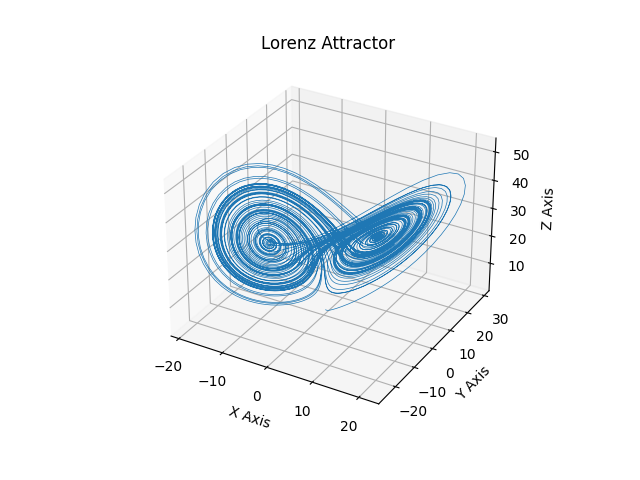# Lorenz attractor#

This is an example of plotting Edward Lorenz's 1963 "Deterministic Nonperiodic Flow" in a 3-dimensional space using mplot3d.

Note

Because this is a simple non-linear ODE, it would be more easily done using SciPy's ODE solver, but this approach depends only upon NumPy.import numpy as np
import matplotlib.pyplot as plt

def lorenz(xyz, *, s=10, r=28, b=2.667):
"""
Parameters
----------
xyz : array-like, shape (3,)
Point of interest in three-dimensional space.
s, r, b : float
Parameters defining the Lorenz attractor.

Returns
-------
xyz_dot : array, shape (3,)
Values of the Lorenz attractor's partial derivatives at *xyz*.
"""
x, y, z = xyz
x_dot = s*(y - x)
y_dot = r*x - y - x*z
z_dot = x*y - b*z
return np.array([x_dot, y_dot, z_dot])

dt = 0.01
num_steps = 10000

xyzs = np.empty((num_steps + 1, 3))  # Need one more for the initial values
xyzs = (0., 1., 1.05)  # Set initial values
# Step through "time", calculating the partial derivatives at the current point
# and using them to estimate the next point
for i in range(num_steps):
xyzs[i + 1] = xyzs[i] + lorenz(xyzs[i]) * dt

# Plot